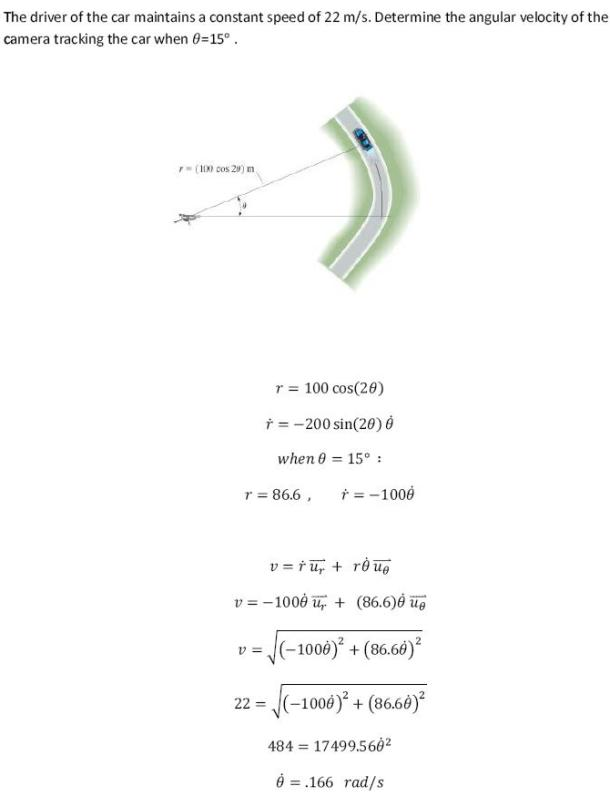dynamics math problems engineering equations dynamics basic mechanics school homework engineering math dynamics formulas dynamics problems dynamics problem solutions to dynamics problems full solution dynamics engineering dynamics problem solution dynamics math problems engineering equations dynamics basic mechanics school homework engineering math dynamics formulas dynamics problems dynamics problem solutions to dynamics problems full solution dynamics engineering dynamics problem solution
dynamics math problems engineering equations dynamics basic mechanics school homework engineering math dynamics formulas dynamics problems dynamics problem solutions to dynamics problems full solution dynamics engineering dynamics problem solution dynamics math problems engineering equations dynamics basic mechanics school homework engineering math dynamics formulas dynamics problems dynamics problem solutions to dynamics problems full solution dynamics engineering dynamics problem solution
Highalphabet Home Page dynamics problem solution dynamics math Highalphabet Statics Lessons
The driver of the car maintains a constant speed of 22 m/s. Determine the angular velocity of the camera tracking the car when theta=15 degrees.The driver of the car maintains a constant speed of 22 m/s. Determine the angular velocity of the camera tracking the car when theta=15 degrees.